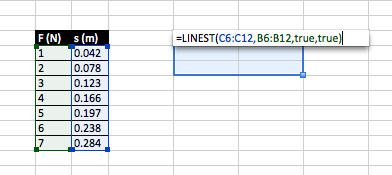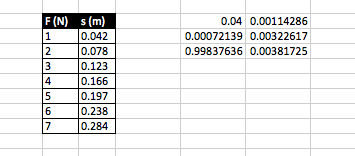by

Finding Standard Error of Slope and Y-Intercept using LINEST in Excel (Linear Regression in Physics Lab)

In Excel, you can apply a line-of-best fit to any scatterplot. The equation for the fit can be displayed but the standard error of the slope and y-intercept are not give. To find these statistics, use the LINEST function instead. The LINEST function performs linear regression calculations and is an array function, which means that it returns more than one value. Let’s do an example to see how it works.

Let’s say you did an experiment to measure the spring constant of a spring. You systematically varied the force exerted on the spring (F) and measured the amount the spring stretched (s). Hooke’s law states the F=-ks (let’s ignore the negative sign since it only tells us that the direction of F is opposite the direction of s). Because linear regression aims to minimize the total squared error in the vertical direction, it assumes that all of the error is in the y-variable. Let’s assume that since you control the force used, there is no error in this quantity. That makes F the independent value and it should be plotted on the x-axis. Therefore, s is the dependent variable and should be plotted on the y-axis. Notice that the slope of the fit will be equal to 1/k and we expect the y-intercept to be zero. (As an aside, in physics we would rarely force the y-intercept to be zero in the fit even if we expect it to be zero because if the y-intercept is not zero, it may reveal a systematic error in our experiment.)

The images below and the following text summarize the mechanics of using LINEST in Excel. Since it is an array function, select 6 cells (2 columns, 3 rows). You can select up to 5 rows (10 cells) and get even more statistics, but we usually only need the first six. Hit the equal sign key to tell Excel you are about to enter a function. Type LINEST(, use the mouse to select your y-data, type a comma, use the mouse to select your x-data, type another comma, then type true twice separated by a comma and close the parentheses. DON’T HIT ENTER. Instead, hold down shift and control and then press enter. This is the way to execute an array function. The second image below shows the results of the function. From left to right, the first row displays the slope and y-intercept, the second row displays the standard error of the slope and y-intercept. The first element in the third row displays the correlation coefficient. I actually don’t know what the second element is. Look it up if you are interested. By the way, you might wonder what the true arguments do. The first true tells LINEST not to force the y-intercept to be zero and the second true tells LINEST to return additional regression stats besides just the slope and y-intercept.1 Comment

1.//

Physics is Phun.